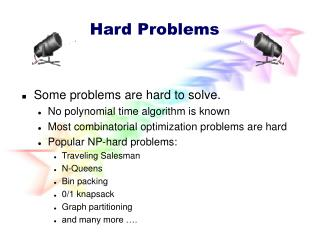# Hard Problems - PowerPoint PPT PresentationDownload PresentationHard Problems

Hard Problems
Download Presentation## Hard Problems

- - - - - - - - - - - - - - - - - - - - - - - - - - - E N D - - - - - - - - - - - - - - - - - - - - - - - - - - -
##### Presentation Transcript

1. Hard Problems Some problems are hard to solve. No polynomial time algorithm is known Most combinatorial optimization problems are hard Popular NP-hard problems: Traveling Salesman N-Queens Bin packing 0/1 knapsack Graph partitioning and many more ….

2. Traveling Salesperson Problem (TSP) • Let Gbe a weighted directed graph. • A tour in Gis a cycle that includes every vertex of the graph. • TSP =>Find a tour of shortest length. • Problem is NP-hard.

3. Home city Visit city Applications Of TSP

4. Applications Of TSP • Tennis practice. • Start with a basket of approximately 200 tennis balls. • When balls are depleted, we have 200 balls lying on and around the court. • The balls are to be picked up by a robot (more realistically, the tennis player). • The robot starts from its station visits each ball exactly once (i.e., picks up each ball) and returns to its station.

5. Robot Station Applications Of TSP

6. Robot Station Applications Of TSP • Manufacturing. • A robot arm is used to drill nholes in a metal sheet. n+1 vertex TSP.

7. 8x8 Chessboard n-Queens Problem A queen that is placed on an n x n chessboard, may attack any piece placed in the same column, row, or diagonal.

8. 8 Queens Problem Place 8 queens on a 8x8 chessboard in such a way that the queens cannot check each other.

9. 4x4 4-Queens Problem Can4 queens be placed on an4 x 4 chessboard so that no queen may attack another queen?

10. 8x8 One possible solution for8-Queens Problem

11. 1 3 5 2 6 4 7 8 8 Queens - Representation Genotype: a permutation of the numbers 1 through 8 Phenotype: a configuration

12. Difficult Problems • Many require you to find either a subset or permutation that satisfies some constraints and (possibly also) optimizes some objective function. • May be solved by organizing the solutionspace into a tree and systematicallysearching this tree for the answer.

13. Permutation Problems • Solution requires you to find a permutation of nelements. • The permutation must satisfy some constraints and possibly optimize some objective function. • Examples. • TSP. • n-queens. • Each queen must be placed in a different row and different column. • Let queen i be the queen that is going to be placed in row i. • Let cibe the column in which queen i is placed. • c1, c2, c3, …, cn is a permutation of [1,2,3, …, n] such that no two queens attack.

14. Solution Space • Permutation problem. • n = 2, {12, 21} • n = 3, {123, 132, 213, 231, 312, 321} • Solution space for a permutation problem has n!members. • Nonsystematic search of the space for the answer takes O(pn!) time, where p is the time needed to evaluate a member of the solution space.

15. Mutation: exchanging two numbers 1 3 5 2 6 4 7 8 1 3 7 2 6 4 5 8 Crossover: combining two parents 1 3 5 2 6 4 7 8 1 3 5 4 2 8 7 6 8 7 6 5 4 3 2 1 8 7 6 2 4 1 3 5 8 Queens - Operators

16. Selection: usinga roulette wheel 1/6 = 17 % fitness(C1) = 1 C3 fitness(C2) = 2 C1 C2 fitness(C3) = 3 2/6 = 33 % 3/6 = 50 % 8 Queens - Fitness & Selection Fitness: penalty of one queen is equal to the number of queens she can check. The fitness of the configuration is equals the sum of the penalties of all queens.